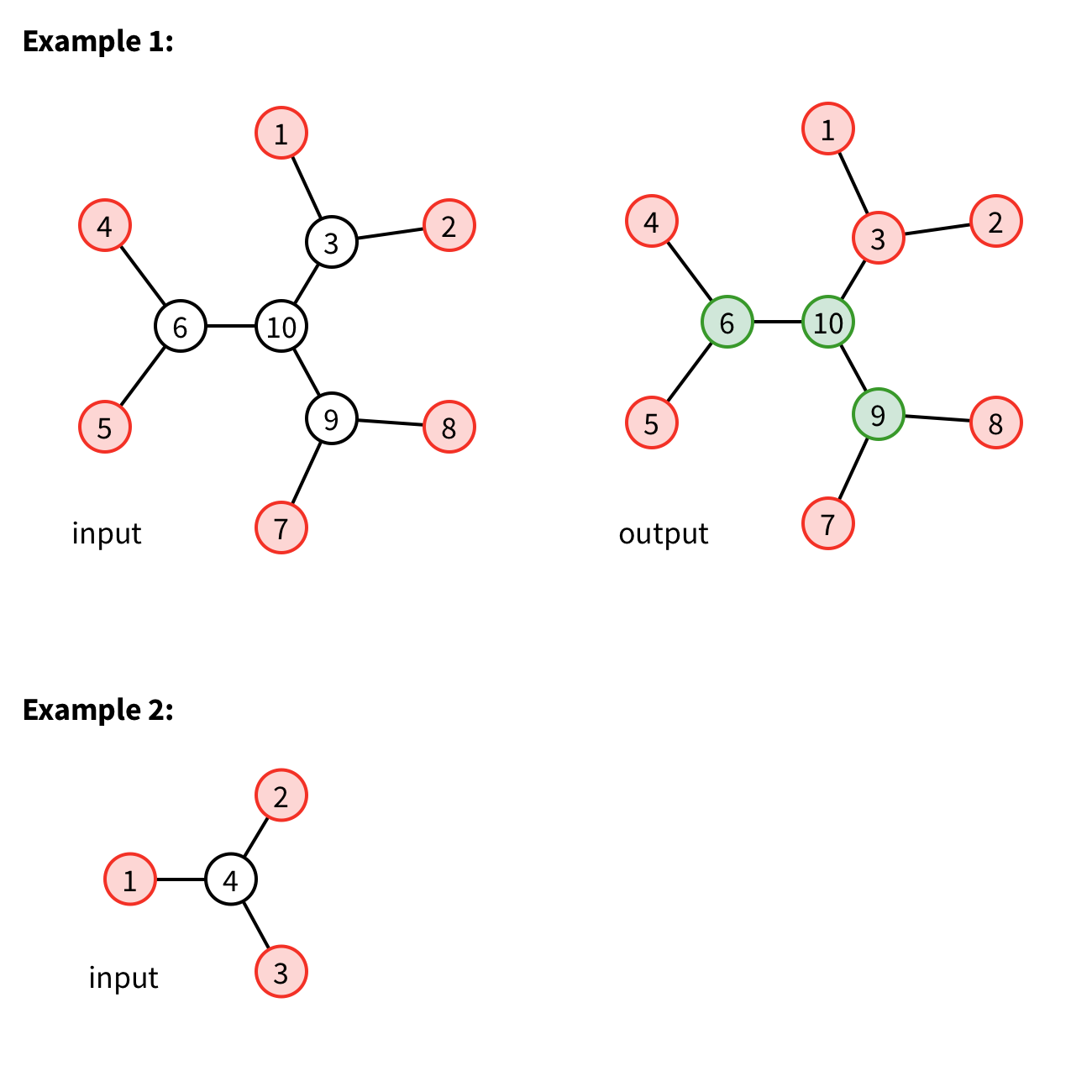Code Submission Evaluation System Login

# E4590 2018 2

 Start: 2018-09-22 13:00:00 End: 2018-09-22 16:00:00

CSES - E4590 2018 2 - Tree coloringCSES - Tree coloring

## Tree coloring

 Time limit: 2.00 s Memory limit: 512 MB

You are given a tree. Each node of the tree has degree either 3 or 1: nodes of degree 3 are called internal nodes and nodes of degree 1 are called leaf nodes. Each leaf node is already colored with one of the colors red and green. Your task is to color each internal node with one of the colors red and green such that the following holds: each internal node has at least one neighbor with color red and at least one neighbor with color green.

Find a valid coloring of internal nodes, or that such coloring does not exist.

Input

The first line of the input contains one number, $n$, the number of nodes. The next $n-1$ lines each contains two numbers, $a$ and $b$, denoting that there exists an edge between nodes $a$ and $b$. Last line of the input contains $n$ numbers, the coloring of each leaf node in order. Number 1 denotes red, 2 green and 0 that the node is an internal node.

Nodes are numbered $1, 2, \ldots, n$.

Output

Output a valid coloring for all nodes, or "IMPOSSIBLE" if no such coloring exists.

Constraints
• $1 \le n \le 10^6$
Example 1

Input:
10 1 3 2 3 4 6 5 6 7 9 8 9 3 10 6 10 9 10 1 1 0 1 1 0 1 1 0 0

Output:
1 1 1 1 1 2 1 1 2 2

Example 2

Input:
4 1 4 2 4 3 4 1 1 1 0

Output:
IMPOSSIBLE